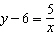Name:    Quiz 3.6-3.8

Multiple Choice
Identify the choice that best completes the statement or answers the question.

1.

Your family is planning to drive 2620 kilometers on a road trip. How many miles is the trip?
 a. 1637.5 miles c. 1455.56 miles b. 4192 miles d. 1871.43 miles

2.

The species Myotis lucifugus is commonly known as the “little brown bat.” An average little brown bat weighs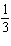ounce. As many as 300,000 little brown bats may share the same roosting location.  How many kilograms would 300,000 little brown bats weigh?
 a. 100,000 kg c. 2812.5 kg b. 13,888.9 kg d. 8437.5 kg

The variables x and y vary directly.  Use the values to write an equation that relates x and y.

3.

y = 27;  x = 9
 a.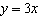c. y =x b.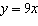d.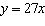4.

Write a direct variation equation that relates x quarts to y liters.
 Length Capacity Weight and Mass 1 in.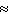2.54 cm1 mi1.6 km 1 qt0.95 L 1 lb0.45kg
 a. x = 0.95y c. y = 0.95x b. y =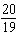x d. y =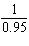x

5.

Write a direct variation equation that relates x kilograms to y pounds.
 Length Capacity Weight and Mass 1 in.2.54 cm1 mi1.6 km 1 qt0.95 L 1 lb0.45kg
 a. y =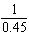x c. x =y b. y = 0.45x d. y =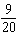x

The variables x and y vary inversely.  Write an equation relating x and y.

6.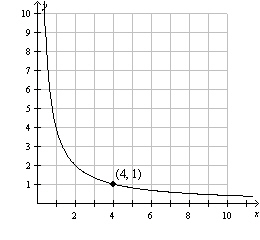a.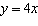c.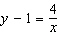b.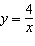d.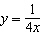7.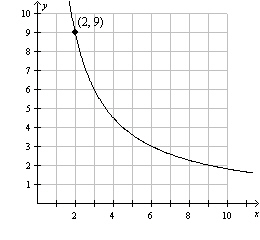a.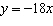c.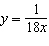b.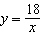d.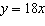Numeric Response

1.

How long is the sea snake in centimeters?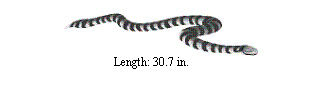Complete the statement using < or >.

1.

29 ft   ?  8 m

Complete the statement.  Round to the nearest hundredth, if necessary.

2.

17 mi?  km

3.

26 mm/sec?  in./sec

Tell whether x and y show direct variation.  Explain your reasoning.

4.

 x 0 1 2 3 y 0 6 12 18

5.

 x –4 –2 0 2 y 4 2 0 2

6.

You rent a scooter for two days while you are on vacation. Tell whether x and y show direct variation. If so, write an equation of direct variation.

 Miles, x 50 30 Cost, y \$30 \$24

Tell whether x and y show direct variation, inverse variation, or neither.  Explain your reasoning.

7.